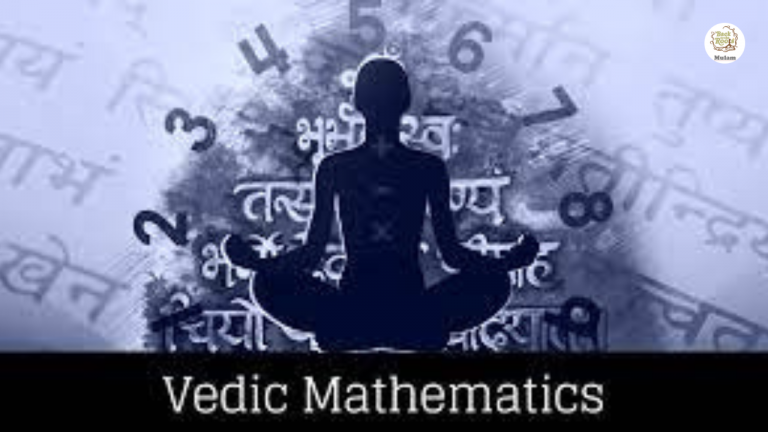Mulam

MULAM – Back to the Roots I Email : connect@mulam.in

## Vedic MathsVedic maths

Vedic mathematics belongs to the Vedic period and was invented in India by a monk named Bharati Krsna Tirthaji between 1911 and 1918. This made maths learning easier, simpler, and faster. This is based on 16 sutras.

These sutras are mentally drafted in their minds or written down on a piece of paper. Students strike themselves if a problem doesn’t get a solution., whereas in Vedic maths, students get the solution and can solve it by themselves in a fraction of a second if they learn this Vedic math. Students enjoy and get excited by doing Vedic math once they start learning. This is the best way to tap into the right side of the brain.

Benefits of Vedic maths

1. It helps in doing simple methods to get a solution, as maths is not a favourite subject of all.
2. Increases ability for numbers: It helps them to solve any calculations like roots, square roots, cubes, and squares in an easier way.
3. It improves concentration and memory: When a child performs calculations mentally, it increases his memory power and concentration.
4. Speed and Accuracy: With these 16 sutras, children can increase their speed in proficiently solving problems.
5. Useful for competitive aspirants: By learning this Vedic math, big calculations, which are time-consuming for aspirants, can be solved at a faster rate and can solve many problems in less time.

Worksheets will help the child solve the problems in a lesser time and more efficient manner.

Importance :

It helps the person solve mathematical problems much more quickly. He can make easy solutions many times faster. for both simple and complex problems. It improves concentration power and a person can memorise difficult concepts in a shorter period. He has scope to develop his skills .

Conclusion:

Maths is not everyone’s favourite subject. Through learning arithmetic, it becomes simpler, easier, and faster. One can also take up a career to improve skills and knowledge, which can be profitable in adverse times or for those who want to take it up as a  second career after marriage or have the determination to improve his/her skills after retirement.

TRICKS

Finding the Square of a Two-Digit Number That Ends with Five

Let us take an example. Imagine you need to find the answer to this: (35) ² =?

Step 135*35* = __25 (the answer will always end with 25)

Step 2: Now, you need to multiply the first digit of the two-digit number by (the first digit plus one to find the term for the blank. In this case, it is 3x (3+1). The answer to this is 4.

Hence the answer to (35 *35) = 1225.

Subtraction from 1000, 10000, 100000 and so on.

This Vedic Maths Subtraction trick is very useful for getting instant subtraction results of any large number from 1000, 1000,… You just keep one formula – Subtract all from 9 and the last from 10.

For example: 1000 – 475 =? (Subtraction from 1000)

We simply subtract each figure in 475 from 9 and the last figure from 10.

9-4=5

9-7=2

10-5=5

So, the answer is 1000 – 475 = 525.